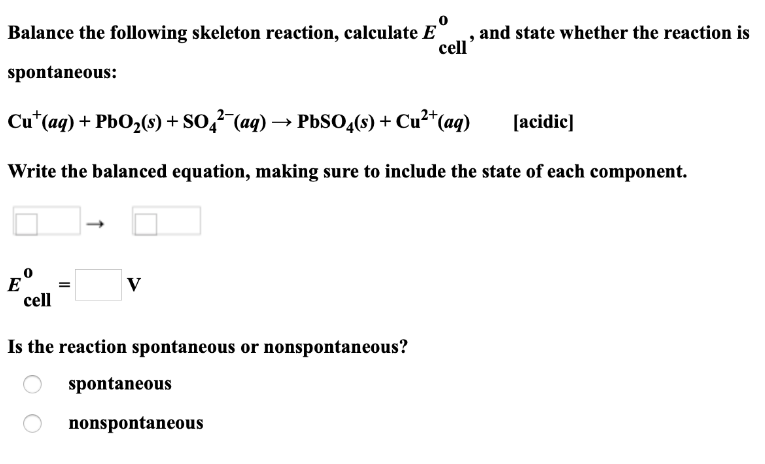# Balance the following skeleton reaction, calculate E°cell, and state whether the reaction is spontaneous: Cu^+(aq) + PbO2(s) + SO4^2-(aq) → PbSO4(s) + Cu^2+(aq) [acidic] Write the balanced equation, making sure to include the state of each component. Is the reaction spontaneous or nonspontaneous?